Next: CORRELATION AND SPECTRA Up: FOURIER AND Z-TRANSFORM Previous: Complex roots

## Inverse Z-transform

Fourier analysis is widely used in mathematics, physics, and engineering as a Fourier integral transformation pair: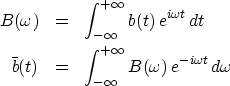(26) (27)
These integrals correspond to the sums we are working with here except for some minor details. Books in electrical engineering redefine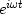as.That is like switchingto.Instead, we have chosen the sign convention of physics, which is better for wave-propagation studies (as explained in IEI). The infinite limits on the integrals result from expressing the Nyquist frequency in radians/second as.Thus, astends to zero, the Fourier sum tends to the integral. When we reach equation (31) we will see that if a scaling divisor of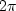is introduced into either (26) or (27), then b(t) will equal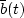.

The Z-transform is always easy to make, but the Fourier integral could be difficult to perform, which is paradoxical, because the transforms are really the same. To make a Z-transform, we merely attach powers of Z to successive data points. When we have B(Z), we can refer to it either as a time function or a frequency function. If we graph the polynomial coefficients, then it is a time function. It is a frequency function if we evaluate and graph the polynomial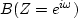for various frequencies.

If the Z-transform amounts to attaching powers of Z to successive points of a time function, then the inverse Z-transform must be merely identifying coefficients of various powers of Z with different points in time. How can this mere identification of coefficients'' be the same as the apparently more complicated operation of inverse Fourier integration? Let us see. The inverse Fourier integral (27) for integer values of time is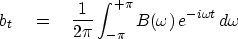(28)
Substituting (21) into (28), we get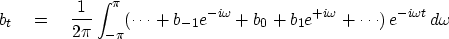(29)
Since sinusoids have as much area above the axis as below, the integration of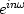over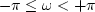gives zero unless n = 0, that is,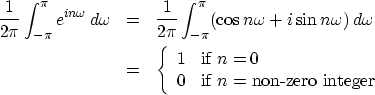(30)
Of all the terms in the integrand (29), we see from (30) that only the term with bt will contribute to the integral; all the rest oscillate and cancel. In other words, it is only the coefficient of Z to the zero power that contributes to the integral, so (29) reduces to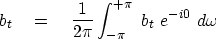(31)
This shows how inverse Fourier transformation is just like identifying coefficients of powers of Z. It also shows why the scale factor in equation (28) is.

## EXERCISES:

1. Let B(Z) = 1 + Z + Z2 + Z3 + Z4. Graph the coefficients of B(Z) as a function of the powers of Z. Graph the coefficients of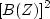.
2. Asmoves from zero to positive frequencies, where is Z and which way does it rotate around the unit circle, clockwise or counterclockwise?
3. Identify locations on the unit circle of the following frequencies: (1) the zero frequency, (2) the Nyquist frequency, (3) negative frequencies, and (4) a frequency sampled at 10 points per wavelength.
4. Given numerical constants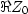and,derive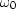and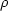.
5. Sketch the amplitude spectrum of Figure 9 from 0 to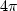.Next: CORRELATION AND SPECTRA Up: FOURIER AND Z-TRANSFORM Previous: Complex roots
Stanford Exploration Project
10/21/1998